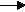gcsescience.com                                       24                                       gcsescience.com

Moles

Masses or Volumes from Electrolysis.

Example 1.

The electrolysis of molten sodium chloride
produces sodium at the cathode and chlorine at the anode.

What mass and volume of chlorine is obtained
if 46 g  of sodium are deposited at the cathode?

Method.
1) Find how many moles of sodium are present in 46 g  of sodium.
RAM of  Na = 23.

moles = mass ÷ RAM

moles = 46 ÷ 23

= 2 moles of sodium.

2)  Write the half equations for the electrolysis.
(i)  Na+  +    e-Na
(ii)  2Cl-  -   2e-Cl2

Balance the half equations,
to give the same number of electrons on each side.
(i)  2Na+  +    2e-2Na
(ii)  2Cl-    -     2e-Cl2

Find the proportion of how many moles of product
are discharged at each electrode.
2 moles of Na at the cathode give 1 mole of
Cl2 at the anode.

3) Find the mass and volume of 1 mole of chlorine.
The mass of 1 mole of
Cl2 is 71 g.
The volume of 1 mole of
Cl2 is 24,000 cm3 (see previous page).

gcsescience.com       The Periodic Table       Index       Moles Quiz       gcsescience.com